Students can download Maths Chapter 6 Trigonometry Additional Questions and Answers, Notes, Samacheer Kalvi 9th Maths Guide Pdf helps you to revise the complete Tamilnadu State Board New Syllabus, helps students complete homework assignments and to score high marks in board exams.

## Tamilnadu Samacheer Kalvi 9th Maths Solutions Chapter 6 Trigonometry Additional Questions

Question 1.
The value of cosec² 60 – 1 is equal to ……..
(a) cos² 60
(b) cot² 60
(c) sec² 60
(d) tan² 60
Solution:
(b) cot² 60

Question 2.
The value of cos 60° cos 30° – sin 60° sin 30° is equal is ……..
(a) cosec 90°
(b) tan 90°
(c) sin 30° + cos 30°
(d) cos 90°
Solution:
(d) cos 90°Question 3.
The value of $$\frac{sin 57°}{cos 33°}$$ is …….
(a) cot 63°
(b) tan 27°
(c) 1
(d) 0
Solution:
(c) 1

Question 4.
If 3 cosec 36° = sec 54° then the value of x is ……..
(a) 0
(b) 1
(c) $$\frac{1}{3}$$
(d) $$\frac{3}{4}$$
Solution:
(c) $$\frac{1}{3}$$

Question 5.
If cos A cos 30° = $$\frac{√3}{4}$$, then the measures of A is ……..
(a) 90°
(b) 60°
(c) 45°
(d) 30°
Solution:
(b) 60°Question 1.
Given Sec θ = $$\frac{13}{12}$$. Calculate all other trigonometric ratios.
Solution:
In the right triangle ABC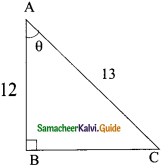BC² = AC² – AB²
= 13² – 12²
= 169 – 144
= 25
∴ BC = $$\sqrt{25}$$
= 5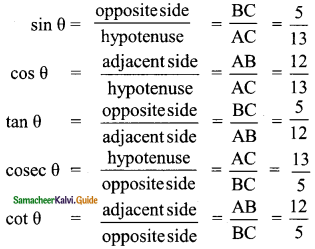Question 2.
If 3 cot A = 4 check weather $$\frac{1- tan²A}{1+ tan²A}$$ = cos² A – sin² A or not?
Solution:
3 cot A = 4
cot A = $$\frac{4}{3}$$
In the right ΔABC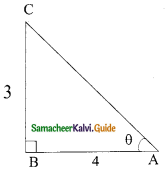AC² = AB² + BC²
= 4² + 3²
= 16 + 9
= 25
= $$\sqrt{25}$$
= 5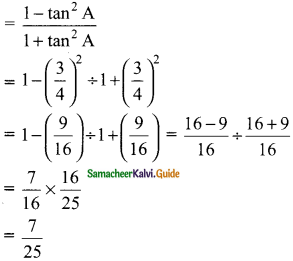Hence $$\frac{1- tan²A}{1+ tan²A}$$ = cos² A – sin² A
R.H.S = cos² A – sin² A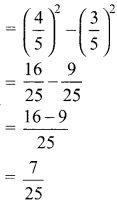L.H.S = R.H.SQuestion 3.
Evaluate $$\frac{sin 30° + tan 45° – cosec 60°}{sec 30° + cos 60° + cot 45°}$$
Solution:
sin 30° = $$\frac{1}{2}$$; tan 45° = 1; cosec 60° = $$\frac{2}{√3}$$; sec 30° = $$\frac{2}{√3}$$; cos 60° = $$\frac{1}{2}$$; cot 45° = 1
$$\frac{sin 30° + tan 45° – cosec 60°}{sec 30° + cos 60° + cot 45°}$$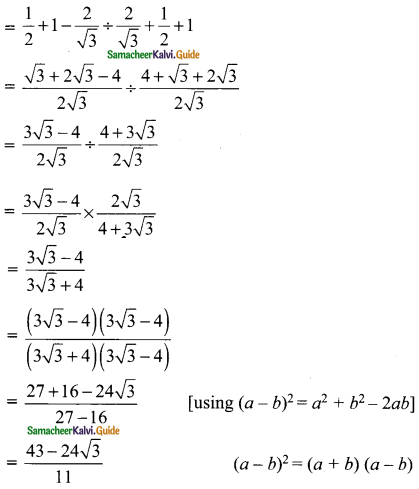The value is $$\frac{43-24√3}{11}$$Question 4.
Find A if sin 20° tan A sec 70° = √3
Solution:
sin 20° . tan A . sec 70° = √3
sin 20° . sec 70° . tan A = √3
sin (90° – 70°). sec 70° . tan A = √3
cos 70° × latex]\frac{1}{cos 70°}[/latex] tan A = √3
tan A = √3
tan A = tan 60°
∴ ∠A = 60°Question 5.
Find the area of the right triangle with hypotenuse 8 cm and one of the acute angles is 57°
Solution:
In the ΔABC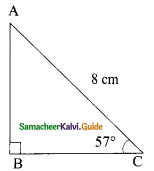sin C = $$\frac{AB}{AC}$$
Sin 57° = $$\frac{AB}{8}$$
0.8387 = $$\frac{AB}{8}$$
∴ AB = 0.8387 × 8
= 0.71 cm
In the ΔABC
cos C = $$\frac{BC}{AC}$$
cos 57° = $$\frac{BC}{8}$$
0.5446 = $$\frac{BC}{8}$$
BC = 0.5446 × 8
= 4.36
Area of the right ΔABC
= $$\frac{1}{2}$$ × AB × BC sq. units
= $$\frac{1}{2}$$ × 6.71 × 4.36 cm²
= 14.62 cm²
Area of the Δ = 14.62 cm²